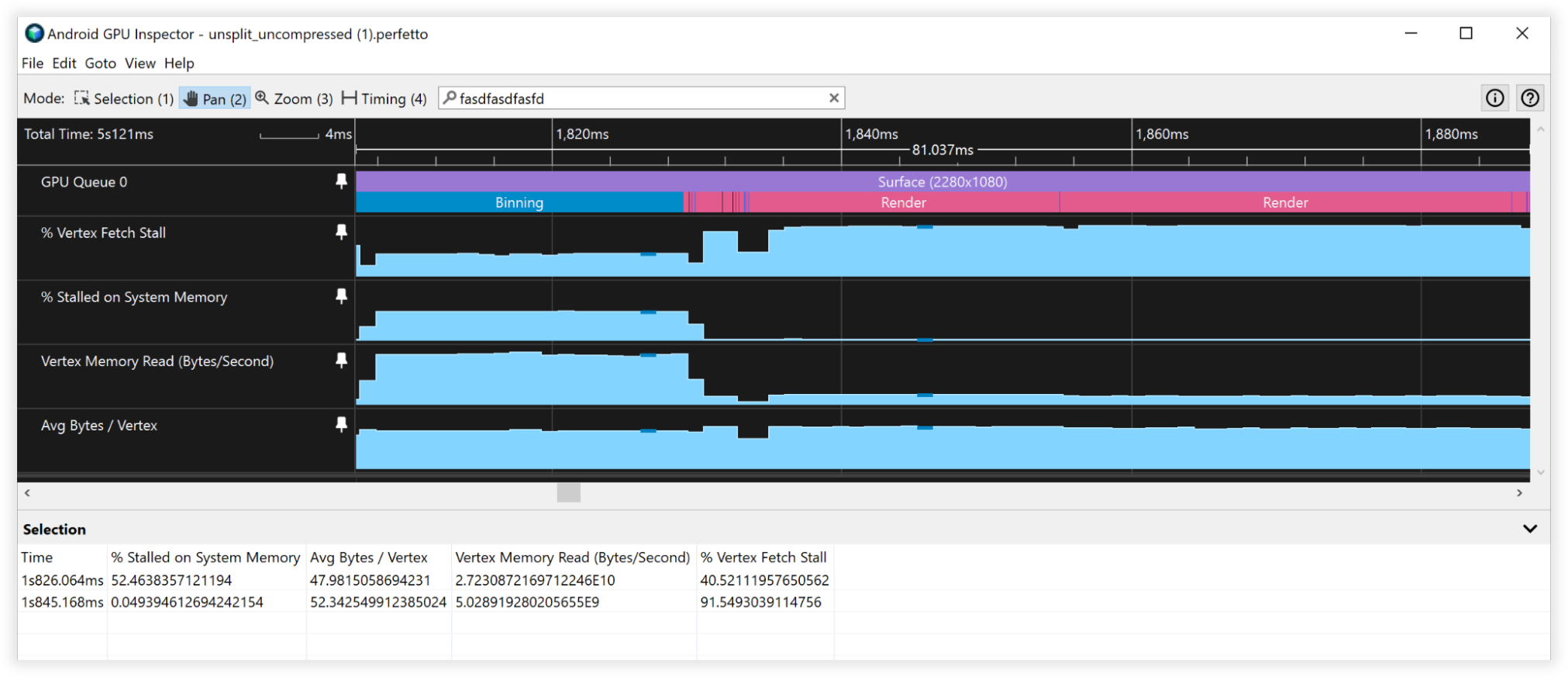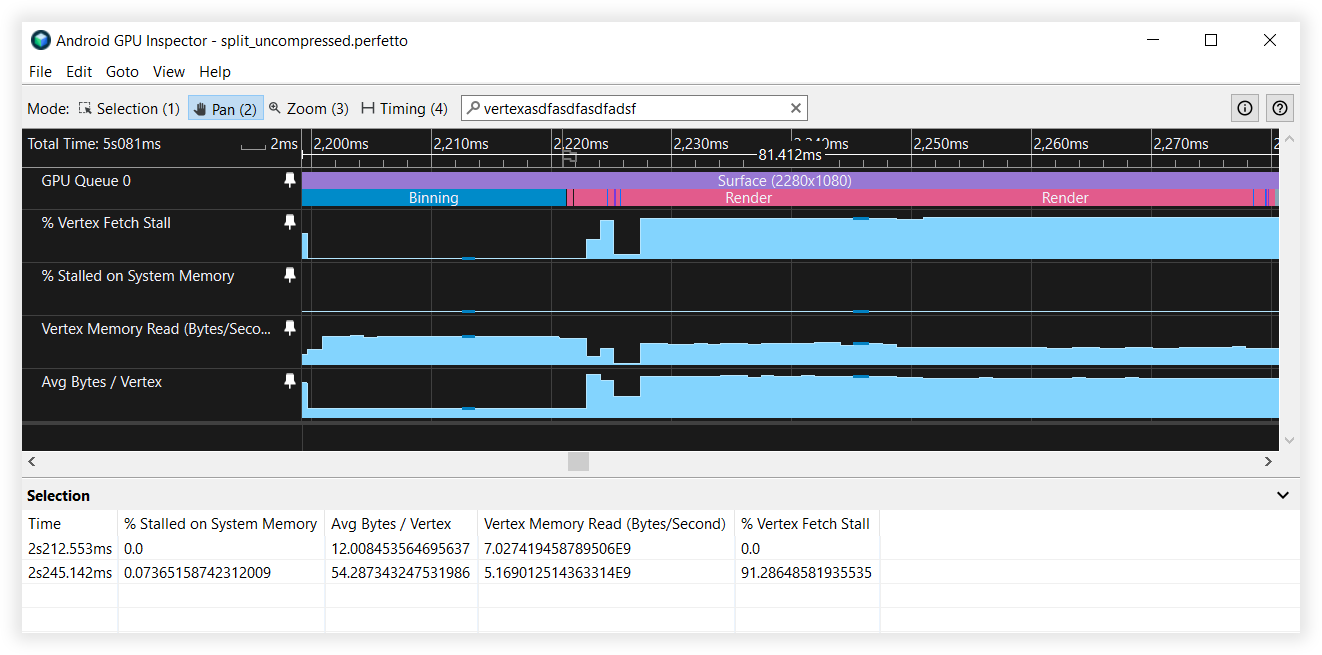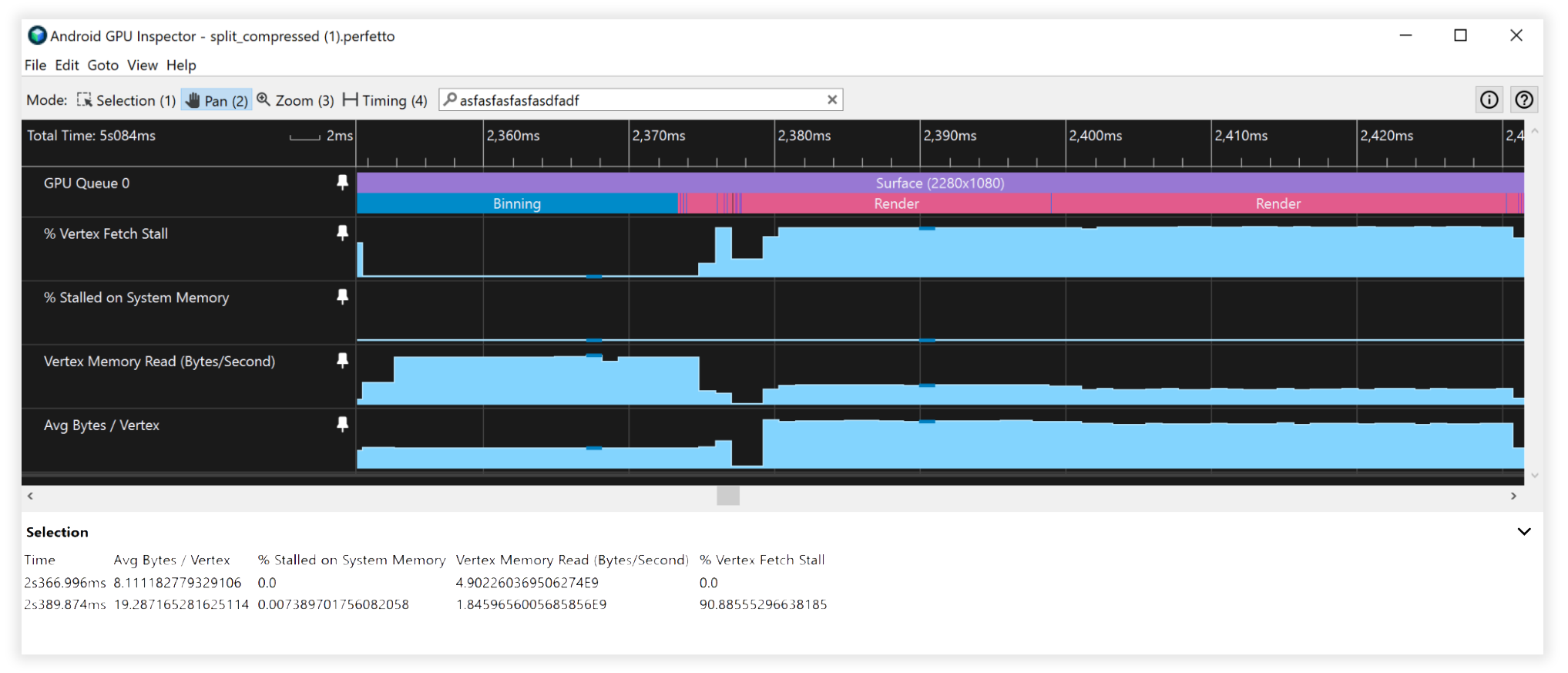# 頂點資料管理

• 頂點壓縮
• 頂點串流分割## 頂點壓縮

• 降低頂點資料屬性的數字精確度 (例如：將 32 位元浮點值降為 16 位元浮點值)
• 代表不同格式的屬性

### 頂點位置

``````uint16_t f32_to_f16(float f) {
uint32_t x = (uint32_t)f;
uint32_t sign = (unsigned short)(x >> 31);
uint32_t mantissa;
uint32_t exp;
uint16_t hf;

mantissa = x & ((1 << 23) - 1);
exp = x & (0xFF << 23);
if (exp >= 0x47800000) {
// check if the original number is a NaN
if (mantissa && (exp == (0xFF << 23))) {
// single precision NaN
mantissa = (1 << 23) - 1;
} else {
// half-float will be Inf
mantissa = 0;
}
hf = (((uint16_t)sign) << 15) | (uint16_t)((0x1F << 10)) |
(uint16_t)(mantissa >> 13);
}
// check if exponent is <= -15
else if (exp <= 0x38000000) {
hf = 0;  // too small to be represented
} else {
hf = (((uint16_t)sign) << 15) | (uint16_t)((exp - 0x38000000) >> 13) |
(uint16_t)(mantissa >> 13);
}

return hf;
}
``````

``````for each position p in Mesh:
p -= center_of_bounding_box // Moves Mesh back to the center of model space
p /= half_size_bounding_box // Fits the mesh into a [-1, 1] cube
vec3<float16> result = vec3(f32_to_f16(p.x), f32_to_f16(p.y), f32_to_f16(p.z));
``````

``````vec3 in in_pos;

void main() {
...
// bounding box data packed into uniform buffer
vec3 decompress_pos = in_pos * half_size_bounding_box + center_of_bounding_box;
gl_Position = proj * view * model * decompress_pos;
}
``````

``````const int BITS = 16

for each position p in Mesh:
p -= center_of_bounding_box // Moves Mesh back to the center of model space
p /= half_size_bounding_box // Fits the mesh into a [-1, 1] cube
// float to integer value conversion
p = clamp(p * (2^(BITS - 1) - 1), -2^(BITS - 1), 2^(BITS - 1) - 1)
``````

### 頂點法向量和切線空間

#### 切線空間

``````const int BITS = 16

quaternion tangent_space_to_quat(vec3 normal, vec3 tangent, vec3 bitangent) {
mat3 tbn = {normal, tangent, bitangent};
quaternion qTangent(tbn);
qTangent.normalize();

//Make sure QTangent is always positive
if (qTangent.w < 0)
qTangent = -qTangent;

const float bias = 1.0 / (2^(BITS - 1) - 1);

//we need to apply a "bias"; while making sure the Quaternion
//stays normalized.
// ** Also our shaders assume qTangent.w is never 0. **
if (qTangent.w < bias) {
Real normFactor = Math::Sqrt( 1 - bias * bias );
qTangent.w = bias;
qTangent.x *= normFactor;
qTangent.y *= normFactor;
qTangent.z *= normFactor;
}

//If it's reflected, then make sure .w is negative.
vec3 naturalBinormal = cross_product(tangent, normal);
if (dot_product(naturalBinormal, binormal) <= 0)
qTangent = -qTangent;
return qTangent;
}
``````

``````for each vertex v in mesh:
quaternion res = tangent_space_to_quat(v.normal, v.tangent, v.bitangent);
// Once we have the quaternion we can compress it
res = clamp(res * (2^(BITS - 1) - 1), -2^(BITS - 1), 2^(BITS - 1) - 1);
``````

``````vec3 xAxis( vec4 qQuat )
{
float fTy  = 2.0 * qQuat.y;
float fTz  = 2.0 * qQuat.z;
float fTwy = fTy * qQuat.w;
float fTwz = fTz * qQuat.w;
float fTxy = fTy * qQuat.x;
float fTxz = fTz * qQuat.x;
float fTyy = fTy * qQuat.y;
float fTzz = fTz * qQuat.z;

return vec3( 1.0-(fTyy+fTzz), fTxy+fTwz, fTxz-fTwy );
}

vec3 yAxis( vec4 qQuat )
{
float fTx  = 2.0 * qQuat.x;
float fTy  = 2.0 * qQuat.y;
float fTz  = 2.0 * qQuat.z;
float fTwx = fTx * qQuat.w;
float fTwz = fTz * qQuat.w;
float fTxx = fTx * qQuat.x;
float fTxy = fTy * qQuat.x;
float fTyz = fTz * qQuat.y;
float fTzz = fTz * qQuat.z;

return vec3( fTxy-fTwz, 1.0-(fTxx+fTzz), fTyz+fTwx );
}

void main() {
vec4 qtangent = normalize(in_qtangent); //Needed because 16-bit quantization
vec3 normal = xAxis(qtangent);
vec3 tangent = yAxis(qtangent);
float biNormalReflection = sign(in_qtangent.w); //ensured qtangent.w != 0
vec3 binormal = cross(normal, tangent) * biNormalReflection;
...
}
``````

#### 僅限法向量``````const int BITS = 8

// Assumes the vector is unit length
// sign() function should return positive for 0
for each normal n in mesh:
float invL1Norm = 1.0 / (abs(n.x) + abs(n.y) + abs(n.z));
vec2 res;
if (n.z < 0.0) {
res.x = (1.0 - abs(n.y * invL1Norm)) * sign(n.x);
res.y = (1.0 - abs(n.x * invL1Norm)) * sign(n.y);
} else {
res.x = n.x * invL1Norm;
res.y = n.y * invL1Norm;
}
res = clamp(res * (2^(BITS - 1) - 1), -2^(BITS - 1), 2^(BITS - 1) - 1)
``````

``````//Additional Optimization: twitter.com/Stubbesaurus/status/937994790553227264
vec3 oct_to_vec(vec2 e):
vec3 v = vec3(e.xy, 1.0 - abs(e.x) - abs(e.y));
float t = max(-v.z, 0.0);
v.xy += t * -sign(v.xy);
return v;
``````

``````const int BITS = 8
const float bias = 1.0 / (2^(BITS - 1) - 1)

// Compressing
for each normal n in mesh:
//encode to octahedron, result in range [-1, 1]
vec2 res = vec_to_oct(n);

// map y to always be positive
res.y = res.y * 0.5 + 0.5;

if (res.y < bias)
res.y = bias;

// Apply the sign of the binormal to y, which was computed elsewhere
if (binormal_sign < 0)
res.y *= -1;

res = clamp(res * (2^(BITS - 1) - 1), -2^(BITS - 1), 2^(BITS - 1) - 1)
``````
``````// Vertex shader decompression
vec2 encode = vec2(tangent_encoded.x, abs(tangent_encoded.y) * 2.0 - 1.0));
vec3 tangent_real = oct_to_vec3(encode);
float binormal_sign = sign(tangent_encode.y);
``````

### 頂點 UV 座標

``````const int BITS = 16

for each vertex_uv V in mesh:
V *= clamp(2^BITS - 1, 0, 2^BITS - 1);  // float to integer value conversion
``````

### 頂點壓縮結果

• 頂點記憶體讀取頻寬：
• 特徵分塊：27GB/s 至 9GB/s
• 算繪：4.5B/s 到 1.5GB/s
• Vertex Fetch Stalls：
• 特徵分塊：50% 至 0%
• 算繪：90% 到 90%
• 平均位元組/頂點：
• 特徵分塊：48B 至 16B
• 算繪：52B 至 18B## 頂點串流分割

``````Before:
|Position1/Normal1/Tangent1/UV1/Position2/Normal2/Tangent2/UV2......|

After:
|Position1/Position2...|Normal1/Tangent1/UV1/Normal2/Tangent2/UV2...|
``````

• 32 位元組快取行 (十分常見的大小)
• 頂點格式包括：
• Position, vec3<float32> = 12 bytes
• Normal vec3<float32> = 12 bytes
• UV coordinates vec2<float32> = 8 bytes
• Total size = 32 bytes

GPU 從記憶體為特徵分塊擷取資料時，將會提取 32 個位元組的快取行來執行。如果沒有頂點串流分割，則只會使用此快取行的前 12 個位元組進行特徵分塊，並在擷取下一個頂點時捨棄其餘的 20 個位元組。使用頂點串流分割時，頂點位置會在記憶體中的相鄰位置，因此當 32 位元組的區塊被提取到快取中時，在必需返回主記憶體擷取更多資料前，實際上會包含 2 個完整的頂點位置以供運作，提升了 2 倍之多！

### 頂點串流分割結果

• 頂點記憶體讀取頻寬：
• 特徵分塊：27GB/s 至 6.5GB/s
• 算繪：4.5GB/s 到 4.5GB/s
• Vertex Fetch Stalls：
• 特徵分塊：40% 至 0%
• 算繪：90% 到 90%
• 平均位元組/頂點：
• 特徵分塊：48B 至 12B
• 算繪：52B 至 52B## 複合結果

• 頂點記憶體讀取頻寬：
• 特徵分塊：25GB/s 至 4.5GB/s
• 算繪：4.5GB/s 到 1.7GB/s
• Vertex Fetch Stalls：
• 特徵分塊：41% 至 0%
• 算繪：90% 到 90%
• 平均位元組/頂點：
• 特徵分塊：48B 至 8B
• 算繪：52B 至 19B## 其他注意事項

### 16 與 32 位元的索引緩衝區資料

• 一律分割/區塊網格，使其與 16 位元索引緩衝區相容 (最多 65536 個不重複頂點)。這樣將有助於行動裝置上已建立索引的算繪，因為擷取頂點資料較為便宜且耗電量較低。

### 不支援的頂點緩衝區屬性格式

• 行動裝置未廣泛支援 SSCALED 頂點格式，且使用時如果嘗試模擬該格式，可能會在未擁有硬體支援的驅動程式中造成效能大量損失。一律使用 SNORM，並承擔可忽略的 ALU 成本以進行解壓縮。
[{ "type": "thumb-down", "id": "missingTheInformationINeed", "label":"Missing the information I need" },{ "type": "thumb-down", "id": "tooComplicatedTooManySteps", "label":"Too complicated / too many steps" },{ "type": "thumb-down", "id": "outOfDate", "label":"Out of date" },{ "type": "thumb-down", "id": "translationIssue", "label":"Translation issue" },{ "type": "thumb-down", "id": "samplesCodeIssue", "label":"Samples / code issue" },{ "type": "thumb-down", "id": "otherDown", "label":"Other" }]
[{ "type": "thumb-up", "id": "easyToUnderstand", "label":"Easy to understand" },{ "type": "thumb-up", "id": "solvedMyProblem", "label":"Solved my problem" },{ "type": "thumb-up", "id": "otherUp", "label":"Other" }]